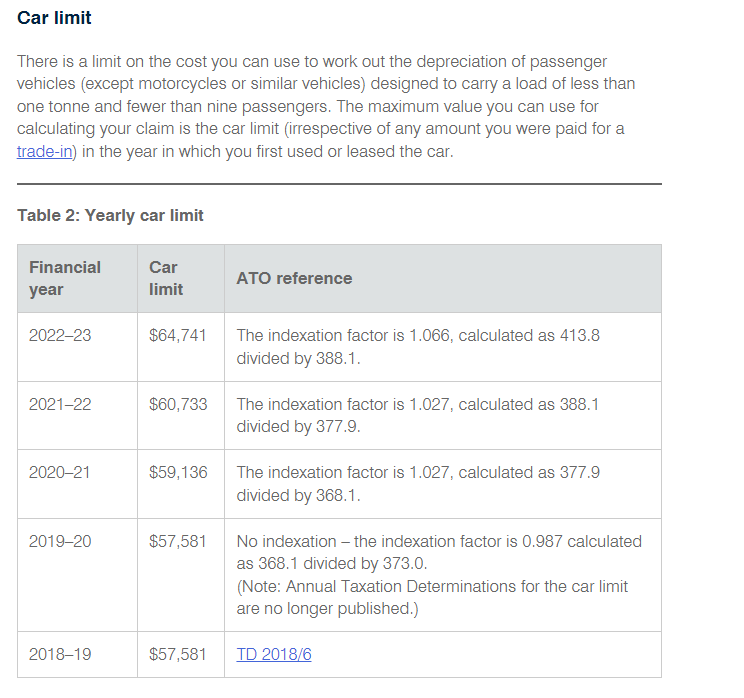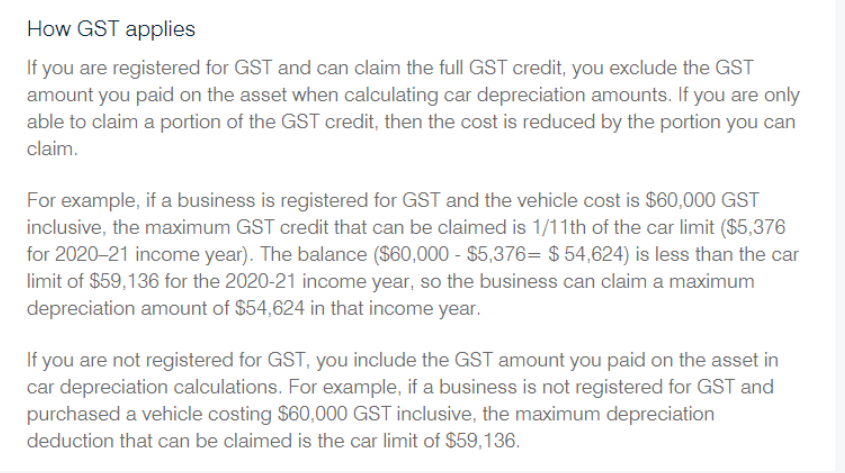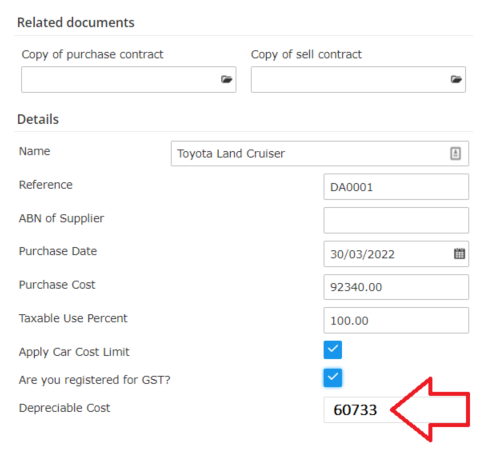Make sure to set up the correct "Category" and "Type" of  Depreciation  -

The car cost depreciation limit is the maximum value that can be assigned to a vehicle for the purpose of a depreciation claim.

Car cost limit information HERE

Cars and Tax 2022-23 HERE

The car limit for 2021-22 is \$60,733. This means that the upper limit on the cost you use to calculate depreciation for the business use is \$60,733 if the car was purchased in the 2021-22 year .

If you then purchase a car, the maximum amount of GST you can claim is one-eleventh of the car limit, in which amount to \$5,521 in the 2021-22 year. This applies if the cost of the car exceeds the car limitExample:

You have to exclude GST from the purchase price first and then compare it with the cost limit. GST is not being excluded from the limit itself.In this example, car cost limit for 2022 is \$60733. The car cost is \$92340.

\$92340 - \$5,521 (GST 1/11th from \$60733) = \$86819 is greater than car cost limit, so the amount will be \$60733.Note: If need to depreciate to 100%, use immediate write off

Here is another example from the ato community support, click HERE to view